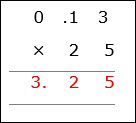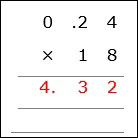# Multiplication of a Decimal by a Whole Number

#### Complete Python Prime Pack

9 Courses     2 eBooks

#### Artificial Intelligence & Machine Learning Prime Pack

6 Courses     1 eBooks

#### Java Prime Pack

9 Courses     2 eBooks

Decimal multiplication is like whole number multiplication.

Rules for Multiplication of a Decimal by a Whole Number

• In the multiplication of a decimal number by a whole number, we first multiply the numbers by ignoring the decimal point.

• We then put the decimal point before as many places in the product as there are in the decimal number.

Multiply 0.13 × 25

### Solution

Step 1:

0.13 × 25

Multiplying without decimal point 13 × 25 = 325

Step 2:

The number of decimal places in 0.13 is two

The number of decimal places in 25 is zero

So the product has two decimal places, i.e., 3.25Multiply 0.24 × 18

### Solution

Step 1:

0.24 × 18

Multiplying without decimal point 24 × 18 = 432

Step 2:

The number of decimal places in 0.24 is two

The number of decimal places in 18 is zero

So the product has two decimal places, i.e., 4.32